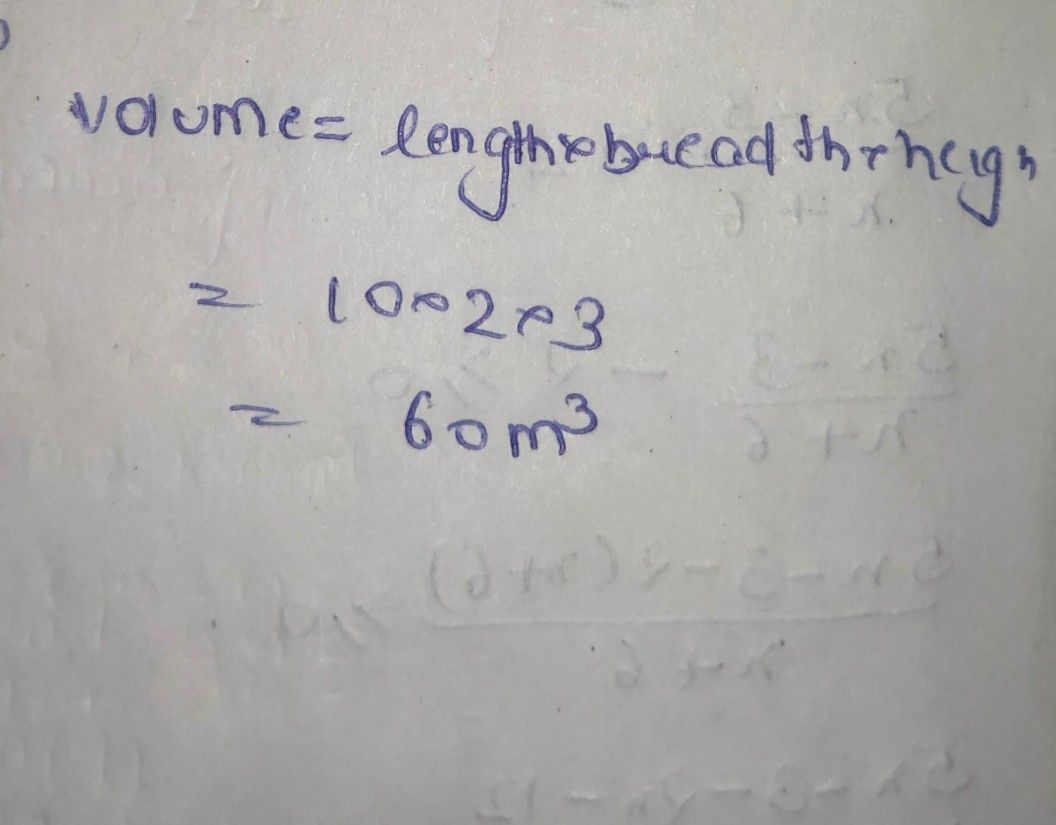Symbol
ProblemUI CU $\left(c\right)$ The figure $\left(i11\right)$ given below show the $cxoss-secHOn$ of a swimming $D^{00}$ 10 m broad, $2mdeCP$ at one end and $3$ m deep at the other end. Calculate the volume $0t$ water it will hold when full, given that $119$ length $15$ $40$ $m$
7th-9th grade
Trigonometry
Search count: 106
SolutionQanda teacher - AskhinaThanks for asking question. You can ask any doubt without any hesitation in direct way also for high quality answers quickly and please rate 5 stars if you are satisfied with my answers.